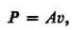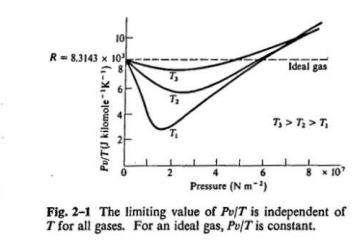### Create an Account

Home / Questions / Estimate as accurately as you can from Fig 2 1 the moll specific volume of co2 at a pressu...

# Estimate as accurately as you can from Fig 2 1 the moll specific volume of co2 at a pressure of 3 107 Nm-1 and a temperature T1 Assume T1 340 K At this pressure and temperature how many

Estimate as accurately as you can from Fig. 2-1 the moll specific volume of co2, at a pressure of 3 x 107 Nm-1 and a temperature T1 Assume T1=340 K. (b) At this pressure and temperature, how many kilo moles of C01 are contained in a tank of volume 0.5 m&#39;? (c) How many kilo moles would the tank contain if CO2 were an ideal gas? 2-3 A cylinder provided with a movable piston contains an ideal gas at a pressure P., specific volume v1, and temperature T1 The pressure and volume are simultaneously increased so that at every instant P and v are related by the equationwhere A is a constant. (a) Express the constant A in terms of the pressure P1, the temperature T1, and the gas constant R. (b) Construct the graph representing the process above in the P-v plane. (c) Find the temperature when the specific volume has doubled, if T1 = 200KMay 08 2020 View more View LessSubscribe To Get Solution